In :
import numpy as np
import pandas as pd

from sktime.forecasting.theta import ThetaForecaster
from sktime.forecasting.ets import AutoETS
from sktime.forecasting.naive import NaiveForecaster
from sktime.forecasting.structural import UnobservedComponents
from sktime.forecasting.exp_smoothing import ExponentialSmoothing
from sktime.forecasting.trend import PolynomialTrendForecaster
from sktime.forecasting.statsforecast import StatsForecastAutoARIMA
from sktime.forecasting.fbprophet import Prophet

from sktime.datasets import load_airline
from sktime.forecasting.base import ForecastingHorizon
from sktime.forecasting.model_selection import temporal_train_test_split
from sktime.performance_metrics.forecasting import mean_absolute_percentage_error
from sktime.utils.plotting import plot_series, plot_correlations, plot_lags

# disable all warnings because they are super annoying
import warnings
warnings.filterwarnings("ignore", category=FutureWarning)


using sktime version 0.11.4

# Demo Time Series Plots in Python¶

## Univariate¶

Model setup. We'll use the sktime load_airline() function to load in the data of international air passengers from 1949-1960 from the classic Box-Jenkins ARIMA paper.

In :
y = load_airline()
y_train, y_test = temporal_train_test_split(y, test_size=12)
fh = ForecastingHorizon(y_test.index, is_relative=False)


You can plot simple diagnostics like the autocorrelation function (ACF) and partial ACF (PACF) with plot_correlations()

In :
# ACF, PACF
plot_correlations(y)

Out:
(<Figure size 864x576 with 3 Axes>,
array([<AxesSubplot:ylabel='Number of airline passengers'>,
<AxesSubplot:title={'center':'Autocorrelation'}>,
<AxesSubplot:title={'center':'Partial Autocorrelation'}>],
dtype=object))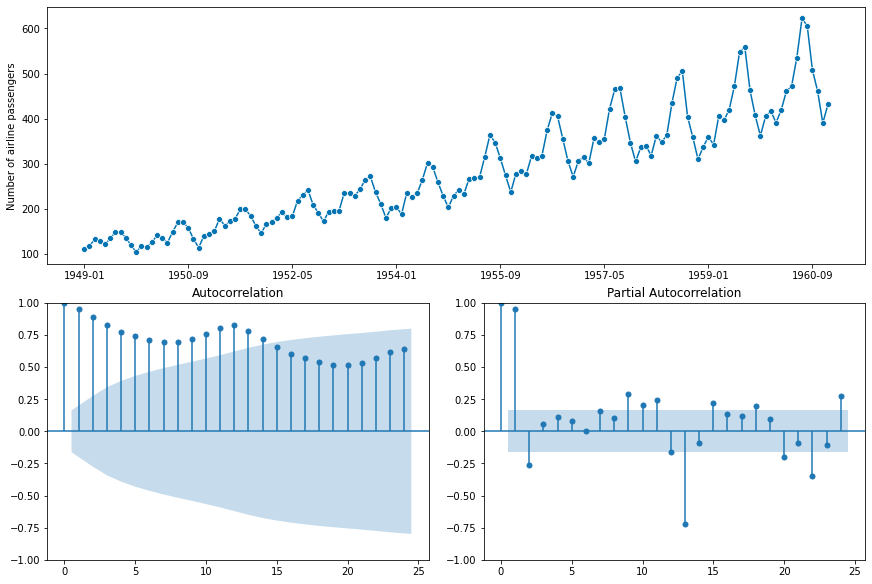In :
# scatter plot of lags vs itself
plot_lags(y, lags=[1,2,3])

Out:
(<Figure size 576x432 with 3 Axes>,
array([<AxesSubplot:xlabel='y(t)', ylabel='y(t + 1)'>,
<AxesSubplot:xlabel='y(t)', ylabel='y(t + 2)'>,
<AxesSubplot:xlabel='y(t)', ylabel='y(t + 3)'>], dtype=object))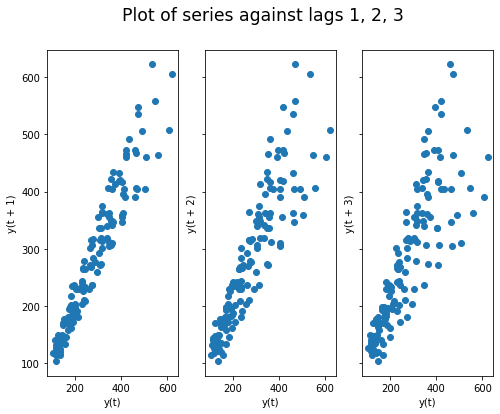## Modeling¶

### Theta Forecaster¶

In :
forecaster = ThetaForecaster(sp=12)  # monthly seasonal periodicity
forecaster.fit(y_train)
y_pred = forecaster.predict(fh)
print("sMAPE: ", mean_absolute_percentage_error(y_test, y_pred, symmetric=True))
plot_series(y, y_pred, labels=["y", "y_pred"])

sMAPE:  0.05231934588593568

Out:
(<Figure size 1152x288 with 1 Axes>,
<AxesSubplot:ylabel='Number of airline passengers'>)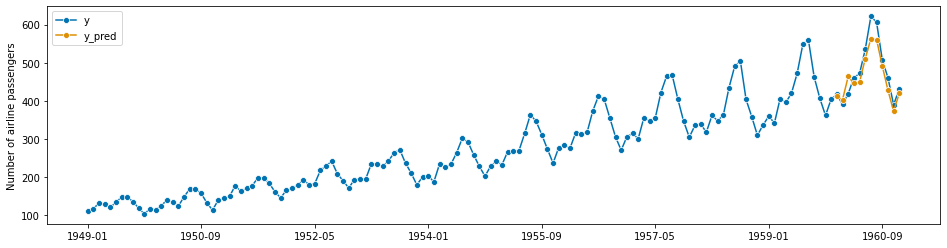Pretty Plot with Prediction Intervals

In :
# Set coverage and then call .predict_interval to return a dataframe with the upper and lower PI bounds
coverage = 0.9
y_pred_ints = forecaster.predict_interval(coverage=coverage)

fig, ax = plot_series(y, y_pred, labels=["y", "y_pred"])
ax.fill_between(
ax.get_lines()[-1].get_xdata(),
y_pred_ints["Coverage"][coverage]["lower"],
y_pred_ints["Coverage"][coverage]["upper"],
alpha=0.2,
color=ax.get_lines()[-1].get_c(),
label=f"{coverage}% prediction intervals",
)
ax.set_title("Theta Forecaster")
ax.legend();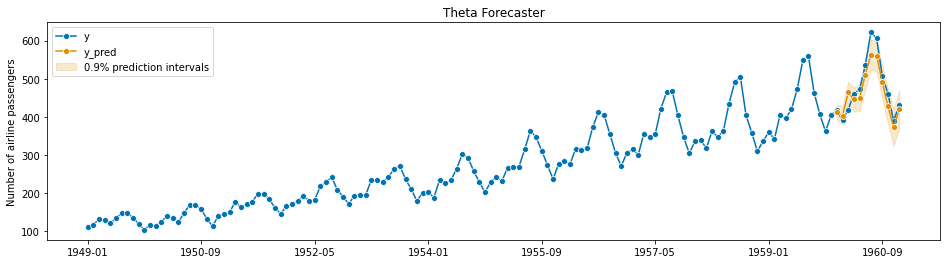Wrap up all the steps above in a function

In :
def run_forecast(y_train, y_test, forecaster, fh, coverage=None, results=True, title=""):
"""Helper function to train a forecaster and plot the results"""
forecaster.fit(y_train)
y_pred = forecaster.predict(fh)
fig, ax = plot_series(y, y_pred, labels=["y", "y_pred"])
if coverage is not None:
# Calculated and add prediction intervals to the plot
y_pred_ints = forecaster.predict_interval(coverage=coverage)
ax.fill_between(
ax.get_lines()[-1].get_xdata(),
y_pred_ints["Coverage"][coverage]["lower"],
y_pred_ints["Coverage"][coverage]["upper"],
alpha=0.2,
color=ax.get_lines()[-1].get_c(),
label=f"{coverage}% prediction intervals",
)
ax.legend();
ax.set_title(title)
print("sMAPE: ", mean_absolute_percentage_error(y_test, y_pred, symmetric=True))
if results:
return forecaster, y_pred


Fitting a linear trendline

In :
forecaster = PolynomialTrendForecaster(degree=1)
run_forecast(y_train, y_test, forecaster, fh, results=False,
title="Linear Trend Forecaster")

sMAPE:  0.12074496965455546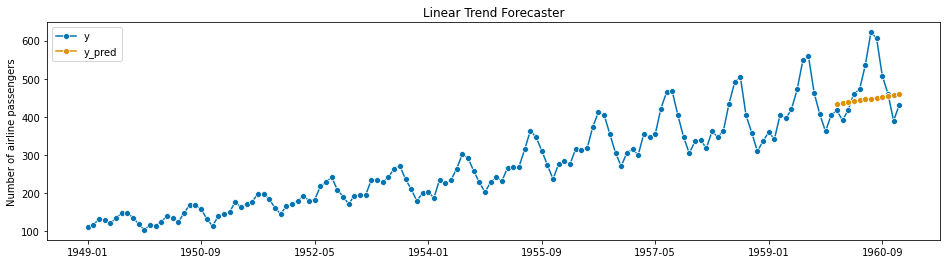In :
forecaster = PolynomialTrendForecaster(degree=2)
run_forecast(y_train, y_test, forecaster, fh, coverage=None, results=False,
title="Polynomial Trend Forecaster (Degree=2)")

sMAPE:  0.11980400632932332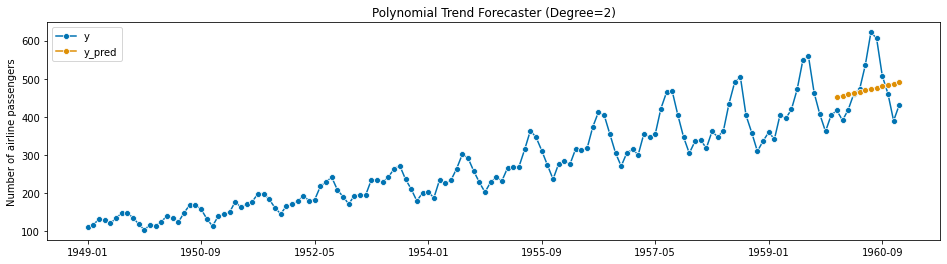Naive Model

In :
forecaster = NaiveForecaster(strategy='last')  # monthly seasonal periodicity
run_forecast(y_train, y_test, forecaster, fh, results=False, title="Naive Model")

sMAPE:  0.16120844670628973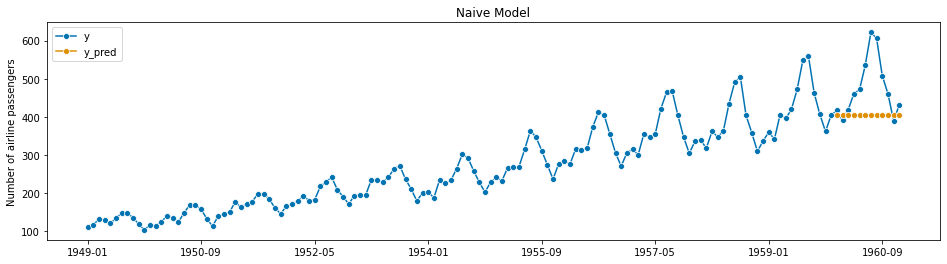Exponential Smoothing (Holt Winters)

In :
forecaster = ExponentialSmoothing()
run_forecast(y_train, y_test, forecaster, fh, coverage=None, results=False,
title="Simple Exponential Smoothing")

sMAPE:  0.16155480390887256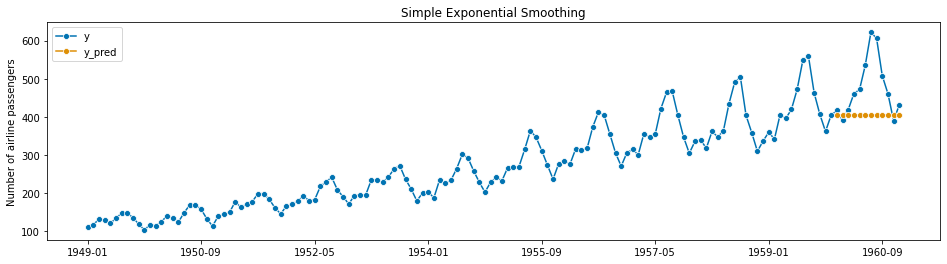In :
forecaster = ExponentialSmoothing(trend='add')
run_forecast(y_train, y_test, forecaster, fh, coverage=None, results=False,
title="Exponential Smoothing with Additive Trend")

sMAPE:  0.14023178895227414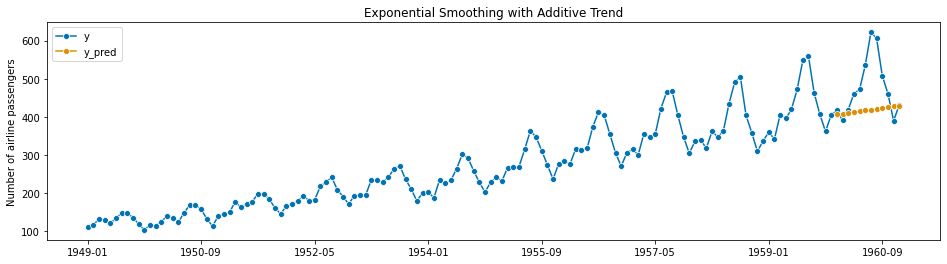In :
forecaster = ExponentialSmoothing(trend='add', seasonal='add', sp=12)
run_forecast(y_train, y_test, forecaster, fh, coverage=None, results=False,
title="Exponential Smoothing with Additive Trend, Additive Seasonality")

sMAPE:  0.02795240869632776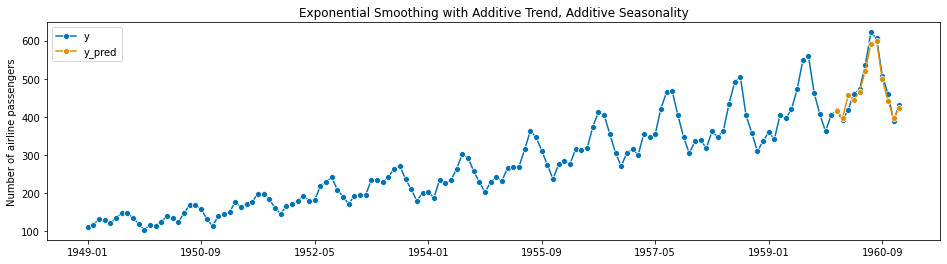AutoETS

In :
forecaster = AutoETS(error='add', trend='add', seasonal='add', sp=12)
run_forecast(y_train, y_test, forecaster, fh, coverage=0.9, results=False,
title="AutoETS (Error, Trend, Seasonality) Model (A,A,A)")

sMAPE:  0.027163603869653663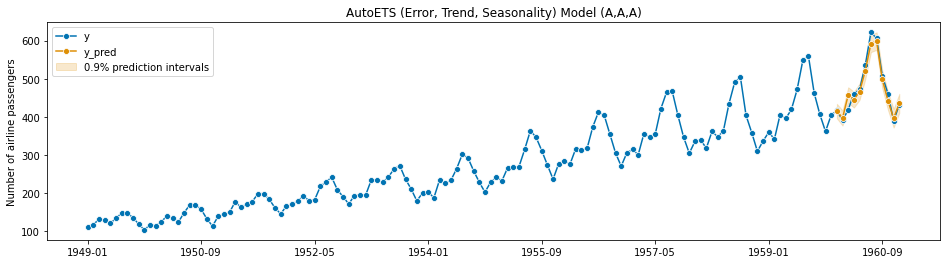StatsForecast AutoARIMA (Box-Jenkins)

In :
forecaster = StatsForecastAutoARIMA(sp=12, d=1, max_p=2, max_q=2)
run_forecast(y_train, y_test, forecaster, fh, coverage=0.9, results=False,
title="AutoARIMA with 12 seasonal periods")

sMAPE:  0.040386441846221005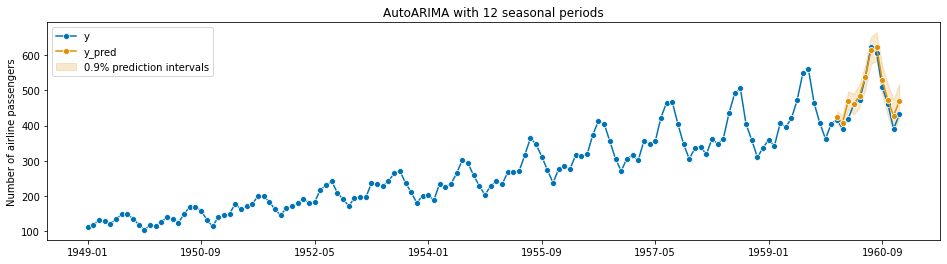In :
forecaster = UnobservedComponents(level="local linear trend", cycle=True, damped_cycle=True, stochastic_cycle=True)
forecaster, y_pred = run_forecast(y_train, y_test, forecaster, fh, coverage=0.9, results=True,
title="Unobserved Components Model")

sMAPE:  0.1472775915337731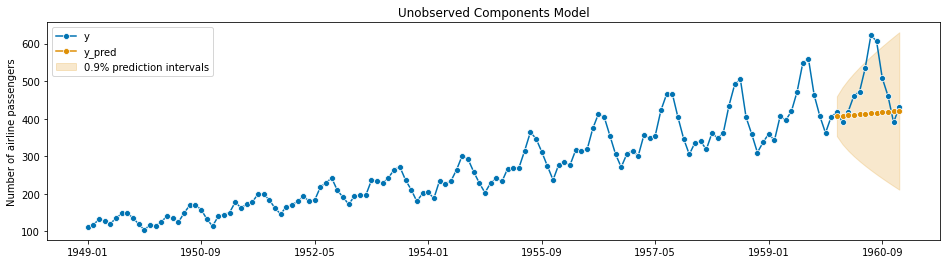In :
forecaster.plot_diagnostics(figsize=(10,9)) # Set the figsize to large for high resolution screens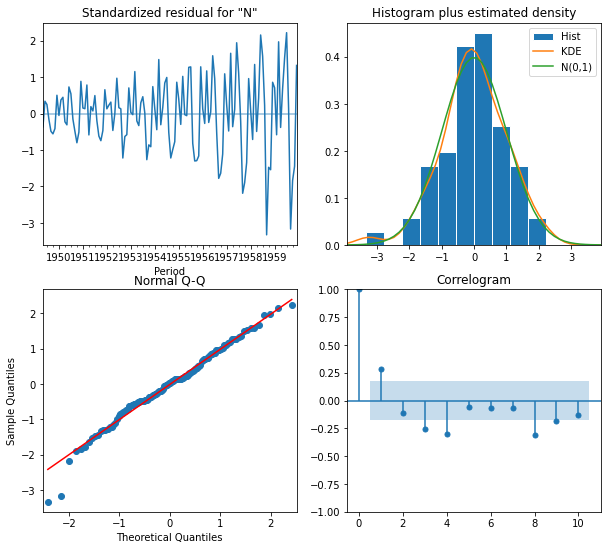In :
forecaster.summary()

Out:
Dep. Variable: No. Observations: Number of airline passengers 132 local linear trend -629.951 + damped stochastic cycle 1271.902 Fri, 03 Jun 2022 1289.014 16:42:19 1278.855 01-31-1949 - 12-31-1959 opg
coef std err z P>|z| [0.025 0.975] 1.241e-06 97.894 1.27e-08 1.000 -191.869 191.869 1013.7659 8218.264 0.123 0.902 -1.51e+04 1.71e+04 1.129e-07 30.479 3.7e-09 1.000 -59.738 59.738 2.056e-05 8187.919 2.51e-09 1.000 -1.6e+04 1.6e+04 0.0436 0.068 0.640 0.522 -0.090 0.177 1.0000 0.088 11.348 0.000 0.827 1.173
 Ljung-Box (L1) (Q): Jarque-Bera (JB): 10.74 5.67 0 0.06 7.91 -0.37 0 3.72

Warnings:
 Covariance matrix calculated using the outer product of gradients (complex-step).

With unobserved components, it's helpful to be able to plot the components...which doesn't appear to be possible in sktime. Below we use statsmodels to plot the results:

In :
import statsmodels.api as sm

# Model specifications

# Unrestricted model, using string specification
unrestricted_model = {
'level': 'local linear trend', 'cycle': True, 'damped_cycle': True, 'stochastic_cycle': True
}

y_train2 = y_train.reset_index()["Number of airline passengers"]

# Output
output_mod = sm.tsa.UnobservedComponents(y_train2, **unrestricted_model)
output_res = output_mod.fit(method='powell', disp=False)

# print(output_res.summary())
fig = output_res.plot_components(legend_loc='lower right', figsize=(15, 15));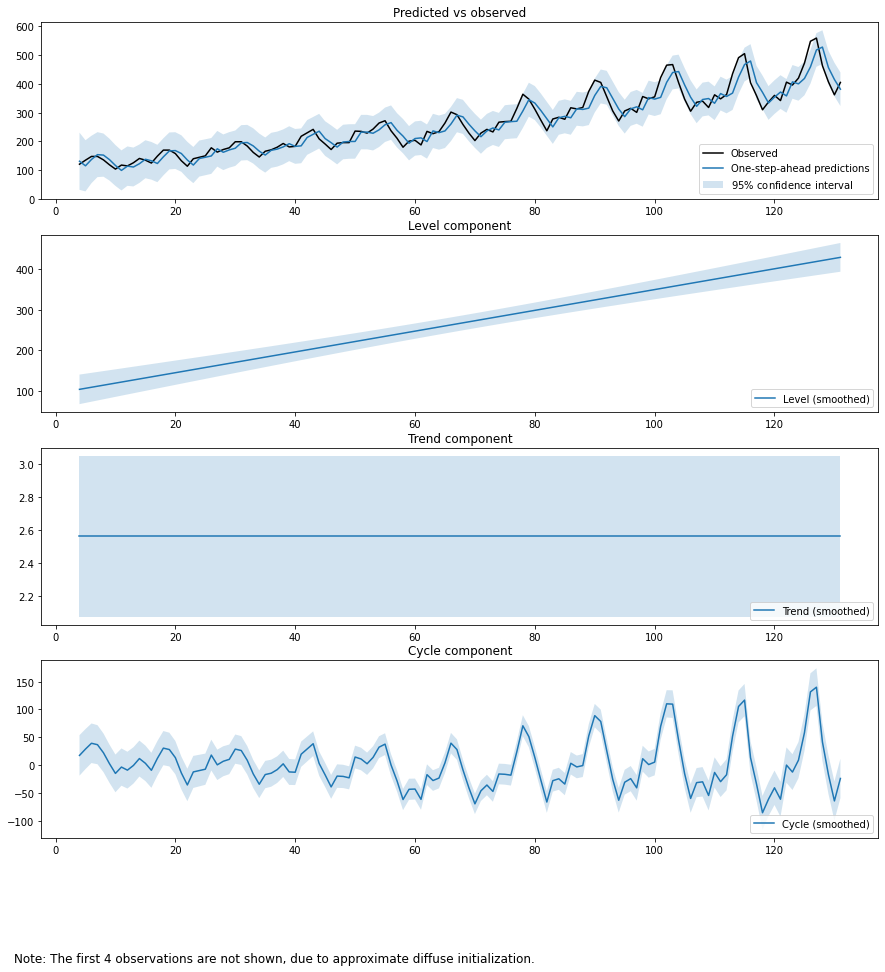And we can calculate the MAPE quickly:

In :
np.mean(np.abs((output_res.predict(start=132, end=132+11).values - y_test.values)/y_test.values))

Out:
0.11036773716103883

### FB Prophet¶

In :
# Prophet requires to have data with a pandas.DatetimeIndex
y_train2, y_test2 = temporal_train_test_split(y, test_size=12)
fh2 = ForecastingHorizon(y_test2.index, is_relative=False)

forecaster = Prophet(
seasonality_mode='multiplicative',
n_changepoints=int(len(y) / 12),
yearly_seasonality=True)
forecaster, y_pred = run_forecast(y_train2, y_test2, forecaster, fh2, coverage=0.9, results=True,
title="FB Prophet Model with Multiplicative Yearly Seasonality")

2022-06-03 16:42:36 prophet INFO: Disabling weekly seasonality. Run prophet with weekly_seasonality=True to override this.
2022-06-03 16:42:36 prophet INFO: Disabling daily seasonality. Run prophet with daily_seasonality=True to override this.

sMAPE:  0.044003264359127055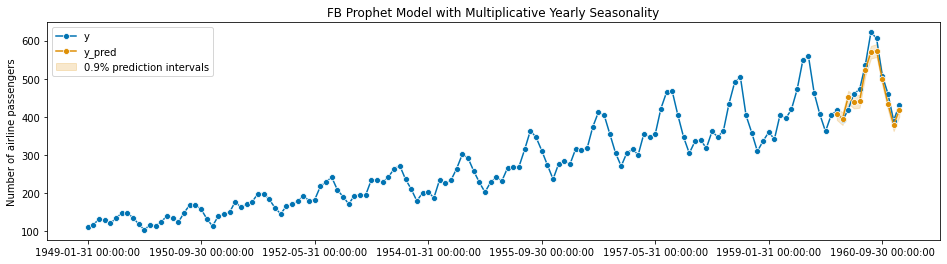In :
# redo y


## Comparing Against a Heuristic Baseline¶

Now let's try a 'linearity' model which predicts quarterly spending based a monthly "percentage of full quarter spending" approach. This is a common approach when predicting how the end of quarter spending is shaping up.

In :
# "Linearity" based on date
y_t = pd.DataFrame(y)
y_t["Q"] = y_t.index.quarter
y_t["YYYYQ"] = y_t.index.year.astype(str) + y_t.index.quarter.astype(str)

# Cumulative Percentage of the Quarter
y_t["cum_pct"] = y_t.groupby(by="YYYYQ")["Number of airline passengers"].transform(lambda x: np.cumsum(x)/sum(x))
y_t["cum_sum"] = y_t.groupby(by="YYYYQ")["Number of airline passengers"].transform(lambda x: np.cumsum(x))
y_t["qtr_total"] = y_t.groupby(by="YYYYQ")["Number of airline passengers"].transform(lambda x: sum(x))

In :
# Find the month, and month in quarter using a mapping dictionary
month_in_qtr = dict()
for j in np.arange(1,4):
for i in range(j,13,3):
month_in_qtr[i] = j
y_t["M"] = y_t.index.month
y_t["Month_in_Qtr"] = y_t["M"].map(month_in_qtr)

In :
# Calculate the Previous 4Q Average Percent of Qtr Spending
y_t["P4Q_avg"] = y_t.groupby("Month_in_Qtr")['cum_pct'].transform(lambda x: x.rolling(4).mean())

In :
y_t.query("Month_in_Qtr==1")["cum_pct"].plot()

Out:
<AxesSubplot:xlabel='Period'>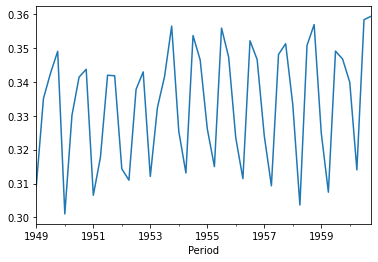In :
# Make the Prediction
y_t["Pred1"] = y_t["cum_sum"]/y_t["P4Q_avg"]

In :
# Calculate accuracy scores and plot the forecasts
subset = y_t.query("Month_in_Qtr==1")
print("MAPE: ", mean_absolute_percentage_error(subset.qtr_total[4:], subset.Pred1[4:], symmetric=False))
print("sMAPE: ", mean_absolute_percentage_error(subset.qtr_total[4:], subset.Pred1[4:], symmetric=True))
# Quarterly Data
plot_series(subset.qtr_total, subset.Pred1, labels=["Actuals", "Pred"])

MAPE:  0.04624210089028735
sMAPE:  0.04644477882368189

Out:
(<Figure size 1152x288 with 1 Axes>, <AxesSubplot:ylabel='qtr_total'>)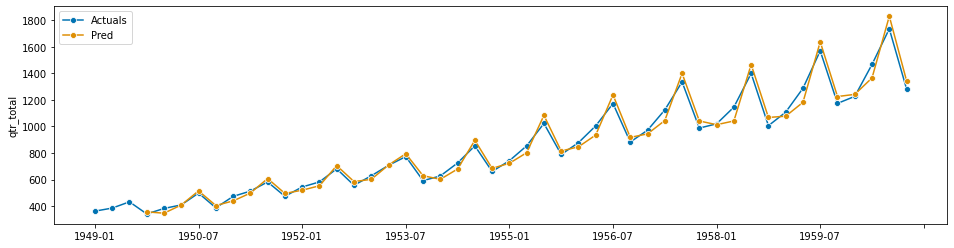In :
subset = y_t.query("Month_in_Qtr==2")
print("MAPE: ", mean_absolute_percentage_error(subset.qtr_total[4:], subset.Pred1[4:], symmetric=False))
print("sMAPE: ", mean_absolute_percentage_error(subset.qtr_total[4:], subset.Pred1[4:], symmetric=True))
# Quarterly Data
plot_series(subset.qtr_total, subset.Pred1, labels=["Actuals", "Pred"])

MAPE:  0.03226663000165359
sMAPE:  0.032058941340971044

Out:
(<Figure size 1152x288 with 1 Axes>, <AxesSubplot:ylabel='qtr_total'>)